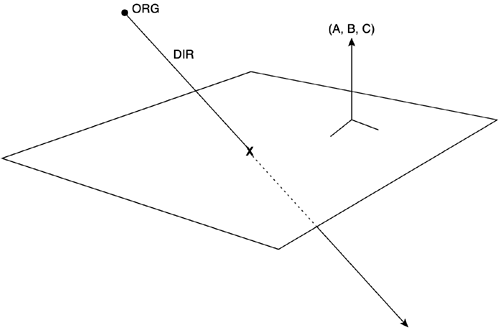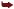# Ray Intersection Tests

Finding which points lie inside which objects is not actually that hard. But its use is limited. The ray intersection test is much more powerful. For example, imagine a fast bullet and a collision detection routine with a relatively small target such as a can of soda. Because the can is relatively small and the bullet is incredibly fast, it could very well happen that on successive frames the bullet is on opposite sides of the soda can, but no single frame exists where the bullet is actually inside the can. Did the intersection happen? The only way to find out is to test the ray formed by the successive positions. If the ray intersected the can, the bullet hit its target.

#### Ray-Plane

Testing for intersection between a ray and a plane is easy (see Figure 22.6). All we have to do is analyze the ray in its parametric form:

` X = org.x + dir.x*t Y = org.y + dir.y*t Z = org.z + dir.z*t `
##### Figure 22.6. Ray-plane intersection test.and the plane with the equation

` AX + BY + CZ + D = 0 `

Half a page of algebra can prove that the preceding equations blend into

` t = - (A*org.x + B*org.y + C*org.z + D) / (A*dir.x + B*dir.y + C*dir.z ) `

Or, if you prefer a more compact notation (using the fact that (A,B,C) is the normal vector to the plane), you can say

` t = - (n·org +D) / (n·dir) `

Obviously, a ray parallel to the vector will return (n·dir)=0, because the dot product of perpendicular vectors equals zero. Thus, we must compute the denominator first, and if it is different from zero, use the numerator to actually compute the t parameter.

Remember that negative t values mean that the ray actually pierces the plane, but not in the direction expressed by the parametric equation of the ray. Actually, if we want the intersection to take place between two specific points in the ray, here is the usual routine:

` compute dir as the vector from one point to the other do not normalize dir use the regular test if the computed t value is in the range from zero to one    the segment intersected the plane .end if `

#### Ray-Triangle

Testing whether a ray intersects a triangle can be performed in a variety of ways. The most popular is not really a test on its own merit, but a composite of two other tests that have already been discussed. The routine would be as follows:

` Compute the intersection between the ray and the support plane for the triangle If there is an intersection point, compute if that point is actually inside the triangleusing a triangle inclusion test. `

Other solutions might be derived using linear algebra, but as far as cost is concerned, none of them offers a significant improvement over this one.

#### Ray-AABB Test

One of the best methods for detecting whether a ray intersects an AABB was introduced by Woo. It uses a three-step algorithm to progressively discard candidate planes, and thus performs costly computations on the minimum possible data set. The pseudocode of the algorithm is as follows:

` From the six planes, reject those with back-facing normals From the three planes remaining, compute the t distance on the ray-plane test Select the farthest of the three distances Test if that point is inside the box's boundaries `

Note that we are assuming we are outside of the object. If we were inside or normals were flipped for some unknown reason, step one would be negated. Thus, the overall test involves

• Six dot products to check the first step

• Three point-ray tests

• A few comparisons for the last step

Incidentally, the 4-step algorithm from the previous section can be used for object-oriented bounding boxes (OOBBs) with minor changes. The first three steps remain unchanged. The last one, however, becomes a bit more complex, as we cannot optimize some computations due to non-axial alignment of the box's support planes. Even so, Woo's algorithm would be a good solution for these cases.

#### Ray-Sphere Test

Let's now analyze the intersection between a ray and a sphere in 3D. Given the ray

` R: X = Rorg.x + Rdir.x * lambda Y = Rorg.y + Rdir.y * lambda Z = Rorg.z + Rdir.z * lambda `

and the sphere defined by

` (X-CenterX)2 + (Y-CenterY)2 + (Z-CenterZ)2 = Radius2 `

the intersection test can fail (if the ray misses the sphere), return one single solution (if the ray touches the sphere tangentially), or return two points (for a general collision). Whichever the case, the preceding equations are easily combined to yield

` A*t2 + B*t + C = 0 `

where

` A = Rdir.x2 + Rdir.y2 + Rdir.z2 B = 2* (Rdir.x2*(Rorg.x-CenterX) + Rdir.y2*(Rorg.y-CenterY) + Rdir.z2*(Rorg.z-CenterZ)) C = (Rorg.x-CenterX)2 + (Rorg.y-CenterY)2 + Rorg.z-CenterZ)2   Radius2 `

In the preceding equation, A usually equals one because the ray's direction vector is normalized, saving some calculations.

Because we have a quadratic equation, all we have to do is solve it with the classic formula

` -b +- sqrt (b2   4AC) / 2a `

The quantity

` B2   4ac `

is referred to as the determinant. If it is negative, the square root function cannot be evaluated, and thus the ray missed the sphere. If it's zero, the ray touched the sphere at exactly one point. If it's greater than zero, the ray pierced the sphere, and we have two solutions, which are

` -b + sqrt (b2   4AC) / 2a -b - sqrt (b2   4AC) / 2a `

#### Ray-Convex Hull

Computing the intersection test between a ray and a convex hull is easy. We loop through the different planes of the convex hull. If we reach the end of the list and all tests were negative (meaning both the first and second point in the ray lie outside the hull), we can stop our search. But what we are interested in are those cases in which a ray's origin has a different sign than the ray's destination. In these cases, we can be sure that a collision took place. Then all we have to do is test the segment and the plane, thus computing the effective intersection point.

#### Ray-General Object (3DDDA)

Computing the intersection point between a ray and a concave object is a complex issue. We can use the Jordan Curve Theorem, but because there can be several intersections, we need additional information in order to decide which one we should return. Thus, a good idea is to use a 3DDDA approach, starting from the cell closest to the origin of the ray and advancing in the direction of the ray. If one cell is OUTSIDE, we move to the next one with no test at all. When we reach the first cell where we get a DON'T KNOW value, we test for the ray with the triangles of the cell. This way the search space is small, and we can converge quickly to the point in space that marks the first collision between the ray and the general object. Again, the speed of the test has a downside of higher memory consumption.Core Techniques and Algorithms in Game Programming2003
ISBN: N/A
EAN: N/A
Year: 2004
Pages: 261